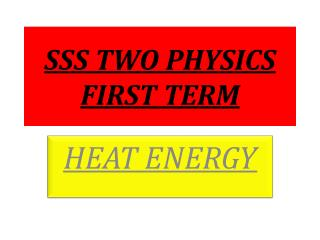DownloadDownload PresentationSSS TWO PHYSICS FIRST TERM

# SSS TWO PHYSICS FIRST TERM

Download Presentation## SSS TWO PHYSICS FIRST TERM

- - - - - - - - - - - - - - - - - - - - - - - - - - - E N D - - - - - - - - - - - - - - - - - - - - - - - - - - -
##### Presentation Transcript

1. SSS TWO PHYSICS FIRST TERM HEAT ENERGY

2. SCHEME OF WORK • 1. MEASUREMENT OF HEAT ENERGY • i) Heat Capacity • ii) Specific Heat Capacity • iii) Determination of Specific Heat Capacities in Solids and Liquids. • 2. LATENT HEAT • i) Concept of Latent Heat • ii) Melting Point and Boiling Point • iii) Specific Latent Heat of Fusion and Vaporization. • iv) Dew Point and Relative Humidity • 3. THE GAS LAWS • i) Boyle’s Law • ii) Charles’ Law • iii) Pressure Law • iv) General Gas Law

3. SCHEME OF WORK • 4. PRODUCTION AND PROPAGATION OF WAVES • i) Pulsating System • ii) Mechanical Waves • iii) Energy Transmitted With Definite Speed, Frequency and Wavelength • iv) Wave-Form • v) Mathematical Relationship • vi) Types of Waves • 5. PROPERTIES OF WAVES • i) Reflection • ii) Refraction • iii) Diffraction • iv) Interference • v) Polarization . 6.CONCEPT OF LIGHT WAVES • i) Source of Light • ii) Propagation of Light • iii) Transmit ion of Light • iv) Sun’s Energy and The Radiation to The Earth

4. SCHEME OF WORK • 7. REFLECTION OF LIGHT AT PLANE SURFACES • i) Laws of Reflection • ii) Formation of Images • iii) Rotation of Mirrors • iv) Applications of Mirror. • 8. REFLECTION OF LIGHT AT CURVED SURFACES • i) Concave and Convex Mirrors • ii) Images Formation on Curved Mirror • iii) Mirror’s Formula • iv) Experimental Determination of Focal Length

5. DEFINITION OF TERMS • Heat capacity is the quantity of heat required to change/raise/lower the temperature of a body by 1K. The unit is Joule per Kelvin. • Specific heat capacity is the quantity of heat required to raise the temperature of a unit mass of a substance through unit temperature. The unit is Joule per Kilogram Kelvin. The formula is Q = mcϴ

6. In calorimetry and method of mixture • Heat lost by substance = heat gained by substance • mcϴ = mcϴ • Specific latent of fusion is the quantity of heat required to convert a unit mass of a substance from solid to liquid without change in temperature. • Mathematically expressed as Q = mL

7. When electrical means of heating is involved, the formula becomes • Ivt = mcϴ • Ivt = mL • Where L is the constant of proportionality called the specific latent heat of fusion or vaporization measured in Joules per kilogram.

8. Definition of terms • Vapour pressure is the pressure exerted by the molecules of a vapour. • Saturated vapour is a vapour that is in contact with its own liquid within a confirmed space. • Saturated vapour pressure(SVP) is the pressure exerted by the saturated vapour. • Dew point is the temperature at which the water vapour present in the air is just sufficient to saturate.

9. Assignment • A block of lead of mass 100kg in a crucible and at a temperature of 40 degree Celsius was placed in an electric furnace rated 10kw. If the melting point of lead is 320 degree Celsius, calculate the • (i) quantity of heat required to heat the lead to its melting point. • (ii) additional heat energy required to melt the lead. • (iii) time taken to supply this additional energy specific heat capacity of lead = 120J/kgk, specific latent heat of fusion of lead = 25000J/kg.